This is “Solving Equations by Factoring”, section 6.6 from the book Beginning Algebra (v. 1.0). For details on it (including licensing), click here.

Has this book helped you? Consider passing it on:
Creative Commons supports free culture from music to education. Their licenses helped make this book available to you.
DonorsChoose.org helps people like you help teachers fund their classroom projects, from art supplies to books to calculators.

## 6.6 Solving Equations by Factoring

### Learning Objectives

1. Verify solutions to quadratic equations.
2. Solve quadratic equations by factoring.
3. Determine a quadratic equation with given solutions.
4. Solve polynomial equations by factoring.

## Solving Quadratic Equations by Factoring

Learning how to solve equations is one of our main goals in algebra. Up to this point, we have solved linear equations, which are of degree 1. In this section, we will learn a technique that can be used to solve certain equations of degree 2. A quadratic equationA polynomial equation with one variable of degree 2. is any equation that can be written in the standard formA quadratic equation written in the form $ax2+bx+c=0.$where a, b, and c are real numbers and $a≠0$. The following are some examples of quadratic equations, all of which will be solved in this section:A solution of a quadratic equation in standard form is called a rootA solution to a quadratic equation in standard form.. Quadratic equations can have two real solutions, one real solution, or no real solution. The quadratic equation $x2+x−6=0$ has two solutions, namely, $x=−3$ and $x=2$.

Example 1: Verify that $x=−3$ and $x=2$ are solutions to $x2+x−6=0$.

Solution: To verify solutions, substitute the values for x and then simplify to see if a true statement results.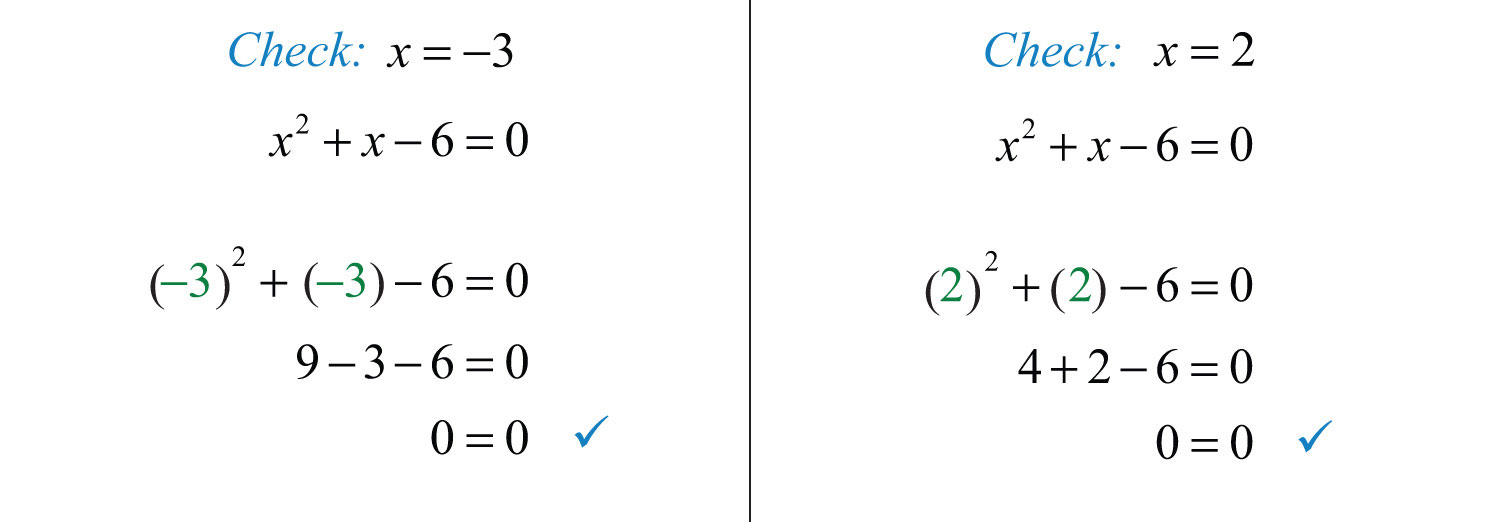Answer: Both values produce true statements. Therefore, they are both solutions to the equation.

Our goal is to develop algebraic techniques for finding solutions to quadratic equations. The first technique requires the zero-product propertyAny product is equal to zero if and only if at least one of the factors is zero.:In other words, if any product is equal to zero, then one or both of the variable factors must be equal to zero.

Example 2: Solve: $(x−8)(x+7)=0$.

Solution: This equation consists of a product of two quantities equal to zero; therefore, the zero-product property applies. One or both of the quantities must be zero.To verify that these are solutions, substitute them for the variable x.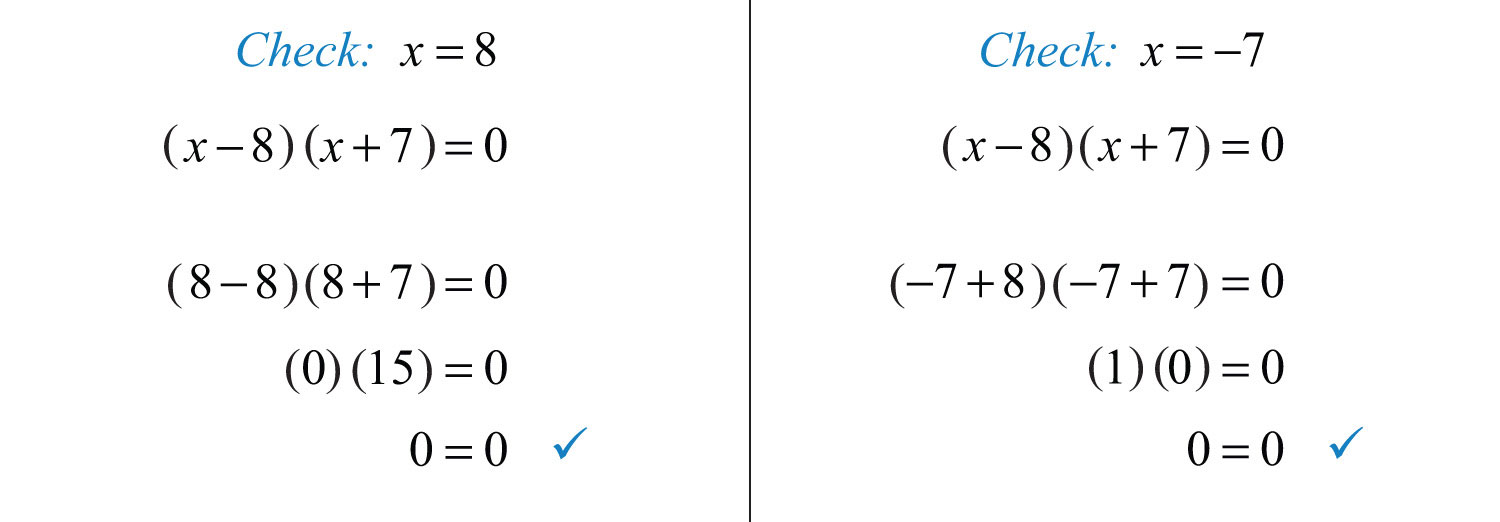Notice that each solution produces a factor that is equal to zero.

Answer: The solutions are 8 and −7.

The quadratic equation may not be given in its factored form.

Example 3: Solve: $x2+3x−10=0$.

Solution: The goal is to produce a product that is equal to zero. We can do that by factoring the trinomial on the left side of the equation.Next, apply the zero-product property and set each factor equal to zero.This leaves us with two linear equations, each of which can be solved for $x$.Check the solutions by substituting into the original equation to verify that we obtain true statements.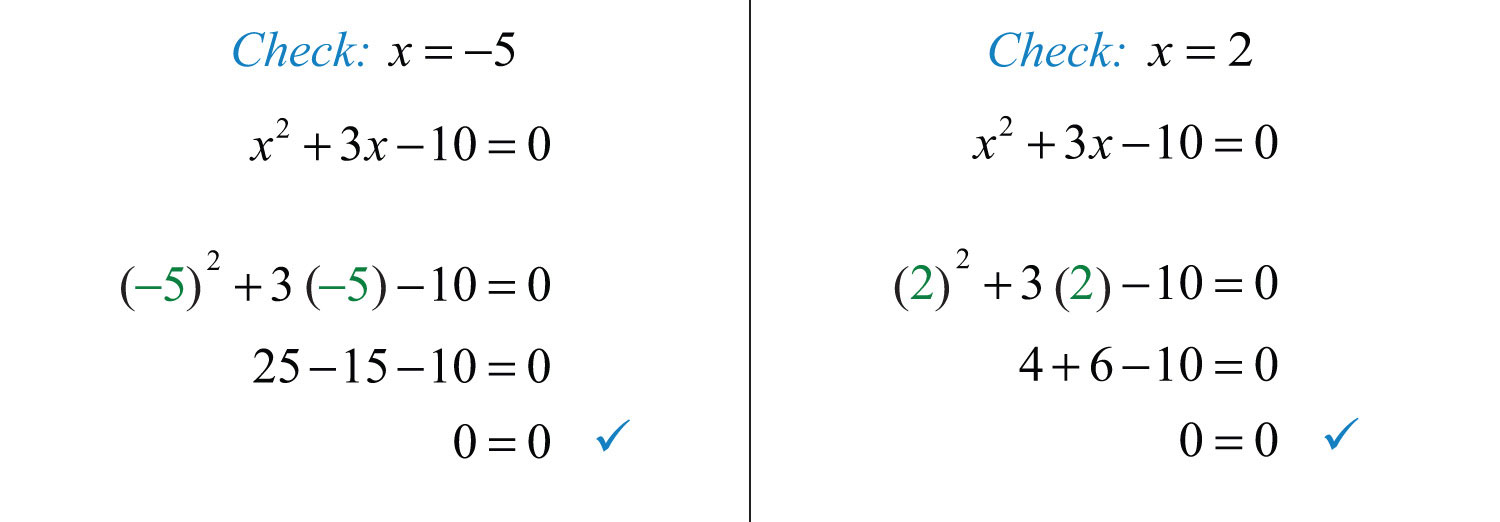Answer: The solutions are −5 and 2.

Using the zero-product property after factoring a quadratic equation in standard form is the key to this technique. However, the quadratic equation may not be given in standard form, and so there may be some preliminary steps before factoring. The steps required to solve by factoringThe process of solving an equation that is equal to zero by factoring it and then setting each variable factor equal to zero. are outlined in the following example.

Example 4: Solve: $2x2+10x+20=−3x+5$.

Solution:

Step 1: Express the quadratic equation in standard form. For the zero-product property to apply, the quadratic expression must be equal to zero. Use the addition and subtraction properties of equality to combine opposite-side like terms and obtain zero on one side of the equation.

In this example, add $3x$ to and subtract 5 from both sides.Step 2: Factor the quadratic expression.Step 3: Apply the zero-product property and set each variable factor equal to zero.Step 4: Solve the resulting linear equations.Answer: The solutions are −5 and −3/2. The check is optional.

Example 5: Solve: $9x2+1=6x$.

Solution: Write this in standard form by subtracting $6x$ from both sides.Once the equation is in standard form, equal to zero, factor.This is a perfect square trinomial. Hence setting each factor equal to zero results in a repeated solution.A repeated solution is called a double rootA root that is repeated twice. and does not have to be written twice.

Try this! Solve: $x2−3x=28$.

Answer: $x=−4$ or $x=7$

### Video Solution

(click to see video)

Not all quadratic equations in standard form are trinomials. We often encounter binomials.

Example 6: Solve: $x2−9=0$.

Solution: This quadratic equation is given in standard form, where the binomial on the left side is a difference of squares. Factor as follows:Next, set each factor equal to zero and solve.Answer: The solutions are 3 and −3, which can also be written as $±3$.

Example 7: Solve: $5x2=15x$.

Solution: By inspection, we see that $x=0$ is a solution to this quadratic equation. Since dividing by zero is undefined, we want to avoid dividing both sides of this equation by x. In general, we wish to avoid dividing both sides of any equation by a variable or an expression containing a variable. We will discuss this in more detail later. The first step is to rewrite this equation in standard form with zero on one side.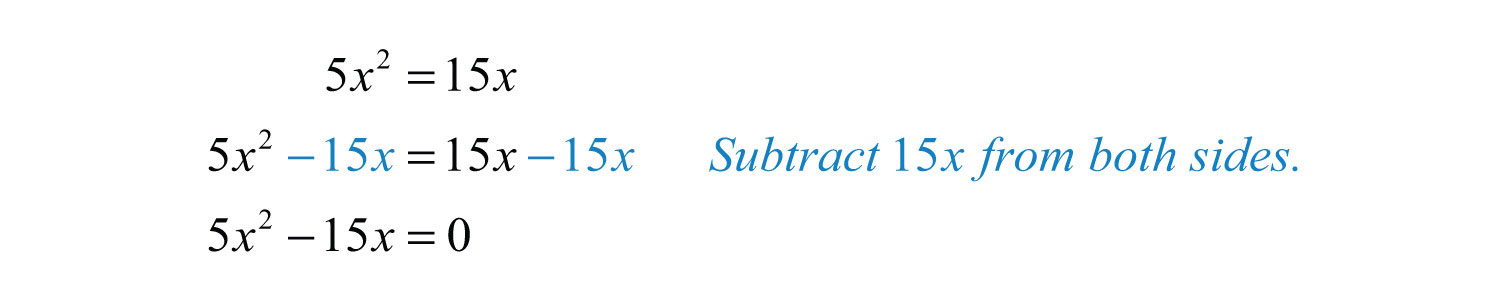Next, factor the expression. Notice that the binomial on the left has a GCF of $5x$.Set each factor to equal to zero.Answer: The solutions are 0 and 3.

Example 8: Solve: $(2x+1)(x+5)=11$.

Solution: This quadratic equation appears to be factored; hence it might be tempting to set each factor equal to 11. However, this would lead to incorrect results. We must rewrite the equation in standard form, equal to zero, so that we can apply the zero-product property.Once it is in standard form, we can factor and then set each factor equal to zero.Answer: The solutions are 1/2 and −6.

Example 9: Solve: $15x2−25x+10=0$.

Solution: We begin by factoring out the GCF of 5. Then factor the resulting trinomial.Next, we set each variable factor equal to zero and solve for x.Notice that the factor 5 is not a variable factor and thus did not contribute to the solution set.

Answer: The solutions are 2/3 and 1.

Example 10: Factor: $52x2+76x−13=0$.

Solution: Clear the fractions by multiplying both sides of the equation by the LCD, which is equal to 6.At this point, we have an equivalent equation with integer coefficients and can factor as usual. Begin with the factors of 15 and 2.The coefficient of the middle term is $7=3(−1)+5(2)$. Factor as follows:Set each factor equal to zero and solve.Answer: The solutions are −2/3 and 1/5.

Try this! Solve: $4x2−9=0$.

### Video Solution

(click to see video)

## Finding Equations with Given Solutions

The zero-product property states,And, in fact, the converse is true as well:When this is the case, we can write the following:We use this property to find equations, given the solutions. To do this, the steps for solving by factoring are performed in reverse.

Example 11: Find a quadratic equation with solutions −7 and 2.

Solution: Given the solutions, we can determine two linear factors.The product of these linear factors is equal to zero when $x=−7$ or $x=2$:Multiply the binomials and present the equation in standard form.Answer: $x2+5x−14=0$. We may check our equation by substituting the given answers to see if we obtain a true statement. Also, the equation found above is not unique and so the check becomes essential when our equation looks different from someone else’s. This is left as an exercise.

Example 12: Find a quadratic equation with integer coefficients, given solutions 1/2 and −3/4.

Solution: To avoid fractional coefficients, we first clear the fractions by multiplying both sides by the denominator.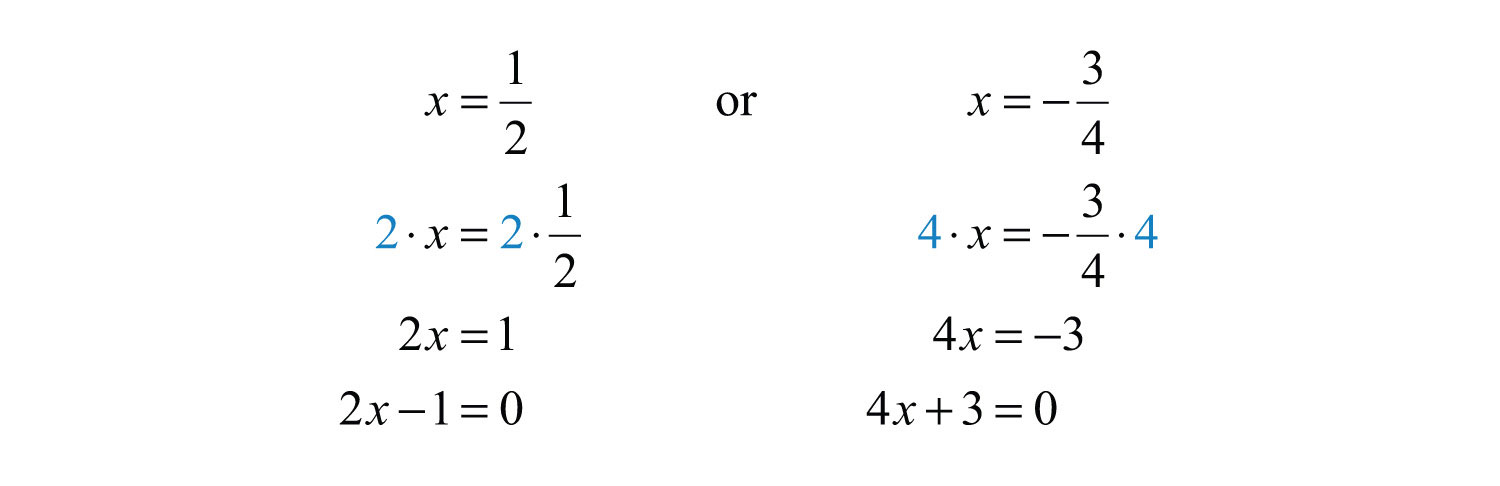Apply the zero-product property and multiply.Answer: $8x2+2x−3=0$

Try this! Find a quadratic equation with integer coefficients, given solutions −1 and 2/3.

Answer: $3x2+x−2=0$

### Video Solution

(click to see video)

## Solving Polynomial Equations by Factoring

The zero-product property is true for any number of factors that make up an equation. If an expression is equal to zero and can be factored into linear factors, then we will be able to set each factor equal to zero and solve for each equation.

Example 13: Solve: $3x(x−5)(3x−2)=0$.

Solution: Set each variable factor equal to zero and solve.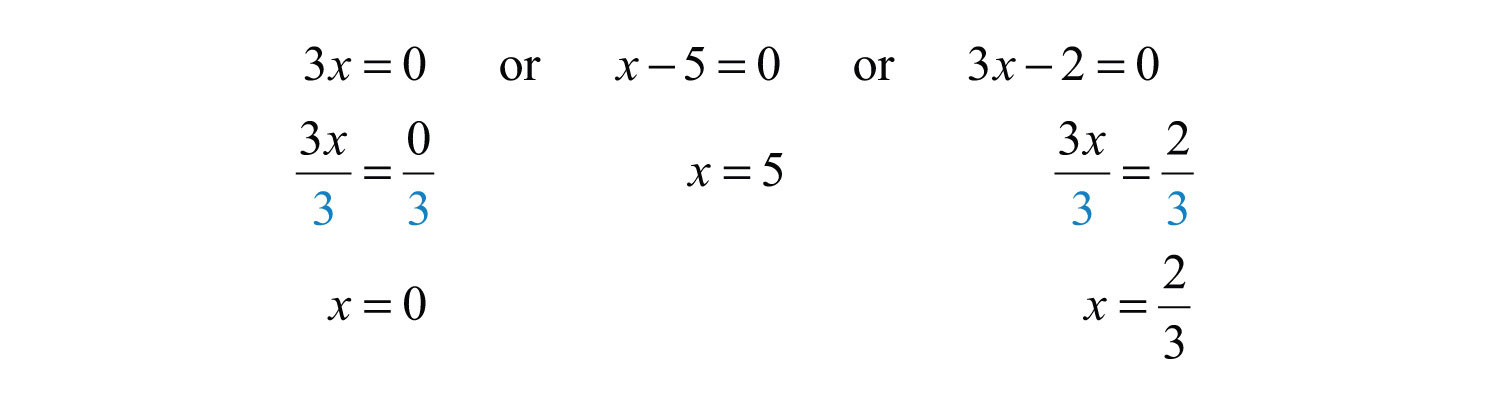Answer: The solutions are 0, 5, and 2/3.

Of course, we cannot expect the equation to be given in factored form.

Example 14: Solve: $x3+2x2−9x−18=0$.

Solution: Begin by factoring the left side completely.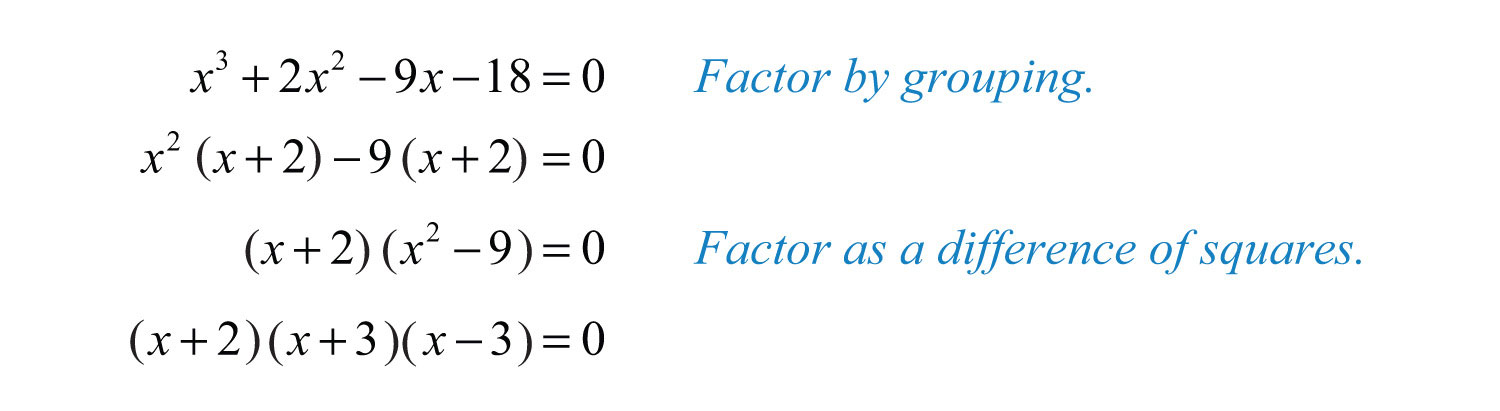Set each factor equal to zero and solve.Answer: The solutions are −2, −3, and 3.

Notice that the degree of the polynomial is 3 and we obtained three solutions. In general, for any polynomial equation with one variable of degree n, the fundamental theorem of algebraGuarantees that there will be as many (or fewer) real solutions to a polynomial with one variable as its degree. guarantees n real solutions or fewer. We have seen that many polynomials do not factor. This does not imply that equations involving these unfactorable polynomials do not have real solutions. In fact, many polynomial equations that do not factor do have real solutions. We will learn how to solve these types of equations as we continue in our study of algebra.

Try this! Solve: $−10x3−18x2+4x=0$.

### Video Solution

(click to see video)

### Key Takeaways

• A polynomial can have at most a number of solutions equal to its degree. Therefore, quadratic equations can have up to two real solutions.
• To solve a quadratic equation, first write it in standard form. Once the quadratic expression is equal to zero, factor it and then set each variable factor equal to zero. The solutions to the resulting linear equations are the solutions to the quadratic equation.
• Not all quadratic equations can be solved by factoring. We will learn how to solve quadratic equations that do not factor later in the course.
• To find a quadratic equation with given solutions, perform the process of solving by factoring in reverse.
• If any polynomial is factored into linear factors and is set to zero, then we can determine the solutions by setting each variable factor equal to zero and solving each individually.

### Topic Exercises

Part A: Solutions to Quadratic Equations

Determine whether the given set of values are solutions to the quadratic equation.

1. {−3, 5}; $x2−2x−15=0$

2. {7, −1}; $x2−6x−7=0$

3. {−1/2, 1/2}; $x2−14=0$

4. {−3/4, 3/4}; $x2−916=0$

5. {−3, 2}; $x2−x−6=0$

6. {−5, 1}; $x2−4x−5=0$

Solve.

7. $(x−3)(x+2)=0$

8. $(x+5)(x+1)=0$

9. $(2x−1)(x−4)=0$

10. $(3x+1)(3x−1)=0$

11. $(x−2)2=0$

12. $(5x+3)2=0$

13. $7x(x−5)=0$

14. $−2x(2x−3)=0$

15. $(x−12)(x+34)=0$

16. $(x+58)(x−38)=0$

17. $(14x+12)(16x−23)=0$

18. $(15x−3)2=0$

19. $−5(x+1)(x−2)=0$

20. $12(x−7)(x−6)=0$

21. $(x+5)(x−1)=0$

22. $(x+5)(x+1)=0$

23. $−2(3x−2)(2x+5)=0$

24. $5(7x−8)2=0$

Part B: Solve by Factoring

Solve.

25. $x2−x−6=0$

26. $x2+3x−10=0$

27. $y2−10y+24=0$

28. $y2+6y−27=0$

29. $x2−14x+40=0$

30. $x2+14x+49=0$

31. $x2−10x+25=0$

32. $3x2+2x−1=0$

33. $5x2−9x−2=0$

34. $7y2+20y−3=0$

35. $9x2−42x+49=0$

36. $25x2+30x+9=0$

37. $2y2+y−3=0$

38. $7x2−11x−6=0$

39. $2x2=−15x+8$

40. $8x−5=3x2$

41. $x2−36=0$

42. $x2−100=0$

43. $4x2−81=0$

44. $49x2−4=0$

45. $x2=4$

46. $9y2=1$

47. $16y2=25$

48. $36x2=25$

49. $4x2−36=0$

50. $2x2−18=0$

51. $10x2+20x=0$

52. $−3x2+6x=0$

53. $25x2=50x$

54. $x2=0$

55. $(x+1)2−25=0$

56. $(x−2)2−36=0$

57. $5x(x−4)=−4+x$

58. $(x−1)(x−10)=22$

59. $(x−3)(x−5)=24$

60. $−2x(x−9)=x+21$

61. $(x+1)(6x+1)=2x$

62. $(x−2)(x+12)=15x$

63. $(x+1)(x+2)=2(x+16)$

64. $(x−9)(2x+3)=2(x−9)$

Clear the fractions by first multiplying both sides by the LCD and then solve.

65. $115x2+13x+25=0$

66. $114x2−12x+37=0$

67. $32x2−23=0$

68. $52x2−110=0$

69. $314x2−212=0$

70. $13x2−15x=0$

71. $132x2−12x+2=0$

72. $13x2+56x−12=0$

73. The sides of a square measure x + 3 units. If the area is 25 square units, then find x.

74. The height of a triangle is 2 units more than its base. If the area is 40 square units, then find the length of the base.

75. The sides of a right triangle have measures that are consecutive integers. Find the length of the hypotenuse. (Hint: The hypotenuse is the longest side. Apply the Pythagorean theorem.)

76. The profit in dollars generated by producing and selling x custom lamps is given by the function $P(x)=−10x2+800x−12000$. How many lamps must be sold and produced to break even? (Hint: We break even when the profit is zero.)

Assuming dry road conditions and average reaction times, the safe stopping distance, d in feet of an average car is given using the formula $d=120v2+v$, where v represents the speed of the car in miles per hour. For each problem below, given the stopping distance, determine the safe speed.

77. 15 feet

78. 40 feet

79. 75 feet

80. 120 feet

Part C: Finding Equations with Given Solutions

Find a quadratic equation with integer coefficients, given the following solutions.

81. −3, 1

82. −5, 3

83. −10, −3

84. −7, −4

85. −1, 0

86. 0, 3/5

87. −2, 2

88. −1/2, 1/2

89. −4, 1/3

90. 2/3, 2/5

91. −1/5, −2/3

92. −3/2, 3/4

93. 3, double root

94. −5, double root

Part D: Solving Polynomial Equations

Solve.

95. $7x(x+5)(x−9)=0$

96. $(x−1)(x−2)(x−3)=0$

97. $−2x(x−10)(x−1)=0$

98. $8x(x−4)2=0$

99. $4(x+3)(x−2)(x+1)=0$

100. $−2(3x+1)(3x−1)(x−1)(x+1)=0$

101. $x3−x2−2x=0$

102. $2x3+5x2−3x=0$

103. $5x3−15x2+10x=0$

104. $−2x3+2x2+12x=0$

105. $3x3−27x=0$

106. $−2x3+8x=0$

107. $x3+x2−x−1=0$

108. $x3+2x2−16x−32=0$

109. $8x3−4x2−18x+9=0$

110. $12x3=27x$

Part E: Discussion Board Topics

111. Explain why $2(x+5)(x−5)=0$ has two solutions and $2x(x+5)(x−5)=0$ has three solutions.

112. Make up your own quadratic equation and post it and the solutions on the discussion board.

113. Explain, in your own words, how to solve a quadratic equation in standard form.

1: Yes

3: Yes

5: No

7: −2, 3

9: 1/2, 4

11: 2

13: 0, 5

15: −3/4, 1/2

17: −2, 4

19: −1, 2

21: −5, 1

23: −5/2, 2/3

25: −2, 3

27: 4, 6

29: 4, 10

31: 5

33: −1/5, 2

35: 7/3

37: −3/2, 1

39: −8, ½

41: −6, 6

43: −9/2, 9/2

45: −2, 2

47: −5/4, 5/4

49: −3, 3

51: −2, 0

53: 0, 2

55: −6, 4

57: 1/5, 4

59: −1, 9

61: −1/2, −1/3

63: −6, 5

65: −3, −2

67: −2/3, 2/3

69: ±7

71: 8

73: 2 units

75: 5 units

77: 10 miles per hour

79: 30 miles per hour

81: $x2+2x−3=0$

83: $x2+13x+30=0$

85: $x2+x=0$

87: $x2−4=0$

89: $3x2+11x−4=0$

91: $15x2+13x+2=0$

93: $x2−6x+9=0$

95: −5, 0, 9

97: 0, 1, 10

99: −3, −1, 2

101: −1, 0, 2

103: 0, 1, 2

105: −3, 0, 3

107: −1, 1

109: −3/2, 1/2, 3/2